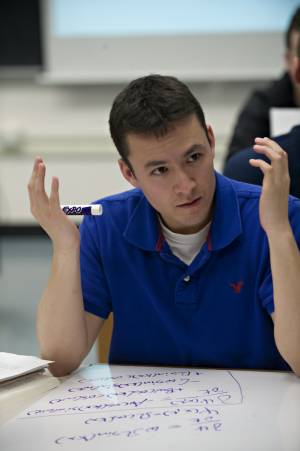Here is a list of surprising things that students don't know:• basis vectors that go with curvilinear coordinates
• matrices as transformations
• power series as approximations
• eigenvectors as the things that are “unchanged” by a transformation
• a geometric conception of fields
• how to add two functions pointwise
• how to read equations as words
• what is planar about plane waves?
• geometric interpretations of dot and cross products
• the meaning of the vertical axis on a graph
• how to shift a graph left or right, up or down
• how to expand a sum (see video from E&E 5/29/09)
• do not understand what “features” are when asked to describe the features of a physical situation

##### Views

New Users

Curriculum

Pedagogy

Institutional Change

Publications

##### Toolbox